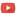KE8QZC | SFW | TSWSyllabus: [pdf] [tex]
Honors syllabus: [pdf] [tex]

Exams
Exam 1: [pdf] [tex]
Exam 2: [pdf] [tex]
Exam 3: [pdf] [tex]

Homework
Homework 1 (due 22 January) (solution) (honors homework: [pdf] [tex]): Section 1.1: #4, 6, 8, 11; Section 1.2: #70, 72, 76, 77, 88, 90, 92, 98, 100, 102, and the following problems:
Problem A: Compute the average value of the function over the given interval from problems #76 and #77.
Problem B: Use the method in the video to estimate $\displaystyle\int_0^{5} e^{-x^2} \mathrm{d}x$ with a rectangle width of $\Delta x = 0.01$. Compare this value to the actual value found from WolframAlpha.
Problem C: Use the method in the video to estimate $\displaystyle\int_0^{5} \sin(t^2) \mathrm{d}t$ with a rectangle width of $\Delta x = 0.01$. Compare this value to the actual value found from WolframAlpha.

Homework 2 (due 28 January) (solution) (honors homework: [pdf] [tex]): Section 1.3: #148, 150, 154, 170, 172, 175, 181, 182; Section 1.5: #262, 264, 266, 272, 274, 276, 281, 282, 292, 294; Section 1.6: #320, 322, 328, 330, 356, 360
Homework 3 (due 4 February) (solution) (honors homework: none this week): Section 2.1: #2, 4, 6, 7, 10, 15, 23; Section 2.2: #74, 77, 79, 82, 83, 86, 90, 91, 99, 101
Homework 4 (due 13 February) (solution) (honors homework: [pdf] [tex]): Section 2.3: #120, 122, 124, 125, 131, 132; Section 2.5: #218, 222, 224, 226, 229, 231
Homework 5 (due 22 February) (solution) (honors homework: [pdf] [tex]): Section 3.1: #6, 10, 12, 14, 20, 21, 22, 38, 42; Section 3.2: #80, 82, 84, 86, 90, 91, 98, 104, 106
Homework 6 (due 27 February) (honors homework: none) (solution): Section 3.3: #134, 135, 136, 137, 138, 139, 141, 142, 148, 151
Homework 7 (due 4 March) (honors homework: [pdf] [tex]) (solution): Section 3.4: #196, 197, 198, 199; Section 3.7: #356, 357, 358, 362, 366, 370, 374, 382
and the following three problems:
Problem A: Compute $\displaystyle\int \dfrac{2x+1}{x^2+4x+4}$.
Problem B: Compute $\displaystyle\int \dfrac{x-1}{(x+1)(x^2+9)}$.
Problem C: Compute $\displaystyle\int \dfrac{1}{(x^2+36)(x^2+4)}$.
Homework 8 (due 27 March) (honors homework: [pdf] [tex]) (solution): Section 5.1: #2, 3, 7, 8, 14, 17, 23, 26, 27, 28; Section 5.2: #67, 70, 72, 73
Homework 9 (due 3 April) (honors homework: [pdf] [tex]) (solution): Section 5.2: #84, 85, 88, 90, 94, 95, 98, 102, 103, 104; Section 5.3: #138, 139, 141, 142, 146, 152, 153, 155, 156, 158, 160, 161, 165, 169, 170
Problem A: Compute $\displaystyle\sum_{n=0}^{\infty} \dfrac{1}{n^2+3n+2}$.
Homework 10 (due 10 April) (honors homework: none) (solution): Section 5.4: #197, 198, 202, 203, 209, 211, 212, 215, 224, 227, 234
Homework 11 (due 15 April) (honors homework: none) (solution): Section 5.5: #250, 251, 252, 254, 261, 263, 265, 282, 283, 304; Section 5.6: #318, 320, 321, 322, 325, 326, 328, 330, 332, 334, 346
Homework 12 (due 3 May) (honors homework: here) (solution): Section 6.1: #13, 14, 15, 16, 18, 19, 23, 24, 33, 35; Section 6.2: #87, 90, 91, 102 (only first sentence), 109; Section 6.3: #116, 119, 120

Quizzes
Quiz 1 (due 25 January) (solution): Compute $\displaystyle\int_{\frac{\pi}{2}}^{\pi} \cos^2(\theta)\sin(\theta) \mathrm{d}\theta$.
Quiz 2 (due 15 February): Compute $\displaystyle\int \sin^2(x) \mathrm{d}x$.
Quiz 3 (due 25 February): Integrate $\displaystyle\int \dfrac{1}{(x-7)(2x+1)} \mathrm{d}x$.
Quiz 4 (due 27 February): Integrate $\displaystyle\int \dfrac{1}{(x^2+1)(x^2+4)} \mathrm{d}x$.
Quiz 5 (due 19 March) (solution): Write the first 5 terms of the sequence defined by $\left\{ \begin{array}{ll} a_{n+1} = a_n + a_{n-1} \\ a_0=2, a_1=1. \end{array} \right.$
Quiz 6 (due 20 March) (solution): Compute the limit $\displaystyle\lim_{n \rightarrow \infty} \dfrac{n^2+3n+1}{5n^2-7n}$.
Quiz 7 (due 26 March) (solution): Compute $\displaystyle\sum_{n=0}^{\infty} \left( -\dfrac{1}{7} \right)^n$.
Quiz 8 (due 8 April) (solution): Does the series $\displaystyle\sum_{n=0}^{\infty} \dfrac{1}{3^n+n}$ converge or diverge? Explain why or why not.
Quiz 9 (due 29 April): Find the Taylor series, centered at $x=0$, of $\cos(x)$.

Notes
Some series for practice (solutions can be found in the Fall 2017 EXAM 4 solution and the Spring 2017 EXAM 4 solutions here)
8 April, alternating harmonic series
8 April, alternating reciprocal of squares
Example 2, 1 April
Example 1, 1 April